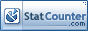### Karakteristik Beton Mutu 16,9 MPa Mengunakan 1% Kawat Bendrat dengan Variasi Panjang

Tri Mulyono

#### Abstract

The research aimed to determine the characteristics of the 16.9 MPa, using steel fibers (bendrat wire) with lenght variations in the concrete mixture 1% by volume-weight of the concrete. This research is an explanatory research with research questions are: how significant is the difference, and what are the characteristics. The total population of each treatment is 12 specimens with a sample of 5 specimens for compressive strength and 4 specimens for split tensile strength for each given treatment which is a random sample, specifically: A (reference concrete); B, C, D, and E for concrete with length variation an expressed as L/D fiber ratio of 50; 62.5; 75 and 87.5. The concrete material meets the standard. At the level of significance 0,05 indicates that the test data are normal distribution and uniform and the characteristics of concrete between reference concrete and concrete fiber different variance values. The unit weight between the reference concrete and the fiber concrete is slightly identical. The slump value decreases with increasing L/D fiber ratio. The concrete characteristics increase up to an L/D fiber ratio of 75. It generates a compressive strength of 19.47% of the design and 16.60% of the reference concrete (17,316 MPa). The split tensile strength is 2,753 MPa (22.29% higher than the design 2,251 MPa) and 18.83% of the reference concrete (2,317 MPa). The flexural strength was 3.638 MPa (18.01% of the 3.083 MPa design) and 1.97% of the reference concrete (3.144 MPa).

#### Keywords

Compressive Strength; Split Tensile Strength; Flexural Strength; Steel Fiber; L/D Ratio

#### Full Text:

PDF

DOI: https://doi.org/10.35334/be.v5i3.2159

Copyright (c) 2021 Borneo Engineering : Jurnal Teknik SipilView MyStat